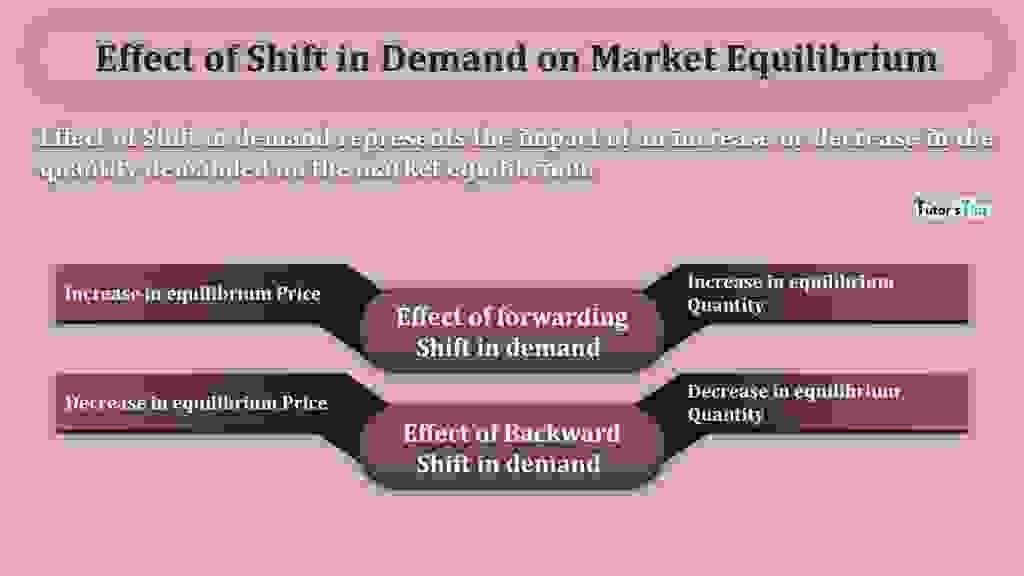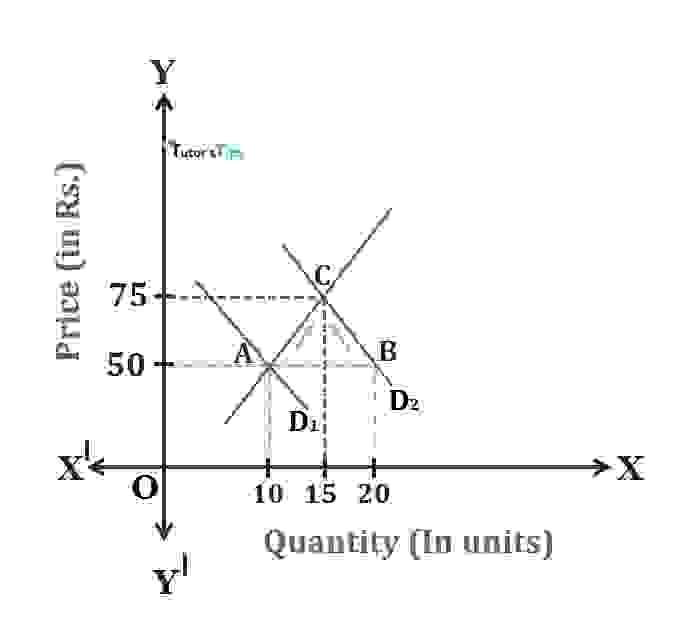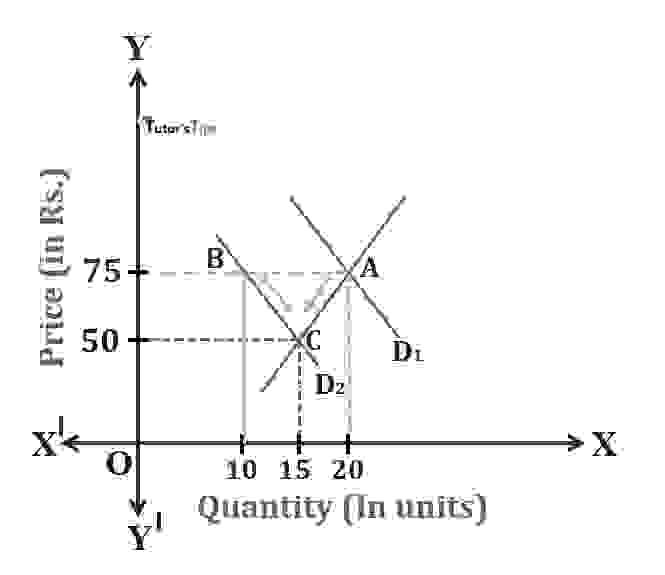# Effect of Shift in Demand on Market EquilibriumThe effect of Shift in demand represents the impact of an increase or decrease in the quantity demanded on the market equilibrium.

The Content covered in this article:

## The shift in the Demand Curve:

It refers to an increase or decrease in demand. It occurs owing to change in determinants of demand other than the commodity’s own price.

For example, the demand increases when the income of consumers increases. Likewise, the demand declines with the reduction in the income level of buyers.

Thus, due to change in any determinants of demand of a commodity other than its own price, the demand curve shifts from its original or initial place. The shift in demand include:

1. Forward Shift
2. Backward Shift

### Effect of forwarding Shift in demand :

The effect of an increase in demand results in an increase in equilibrium price and an increase in equilibrium quantity.

Suppose, When the price of a commodity is Rs.50, the equilibrium quantity is initially 10 units where demand and supply are equal. Also, point A represents the initial equilibrium point. And, if the income of consumers increases for any reason. As a result, the demand curve shifts from D1 to D2. Consequently, the consumers’ demand for commodity increases to 20 units in the market which results in shiting from quantity demanded from point A to B.Effect of increase in demand

As an immediate effect of the increase in demand, there would be excess demand in the market i.e. AB. Owing to the pressure of demand, the price of the commodity tends to increase. Thus, it results in a rise in price to Rs.75. As price increases, the demand contracts as per the law of demand. Thus, the quantity demanded decreases to 15 units from 20 units. Also, as the price increases, the supply also tends to increase, it occurs from 10 units to 15 units.

Thus, the process of extension of supply and contraction of demand continues till the excess demand is fully eliminated and the market clears itself.

Therefore, here,

C point is the new equilibrium point where demand and supply are equal. and, Corresponding to this, the equilibrium quantity is 15 units and the equilibrium price is Rs.75.

hence, the net effect of an increase in demand is:

1. Increase in equilibrium price
2. Increase in equilibrium quantity.

### Effect of backward Shift :

The effect of a decrease in demand results in a decrease in equilibrium price and a decrease in equilibrium quantity.

Suppose, When the price of a commodity is Rs.75, the equilibrium quantity is initially 20 units where demand and supply are equal. Also, point A represents the initial equilibrium point. And, if the income of consumers decreases for any reason. As a result, the demand curve shifts from D1 to D2. Consequently, the consumers’ demand for a commodity decreases to 10 units in the market which results in shiting from quantity demanded from point A to B.Effect of decrease in demand

As an immediate effect of the decrease in demand, there would be excess supply in the market i.e. AB. Owing to the pressure of supply, the price of the commodity tends to decrease. Thus, it results in a fall in price to Rs.50. As price decreases, the demand extends as per the law of demand. Thus, the quantity demanded increases to 15 units from 10 units. Also, as the price decreases, the supply also tends to contracts, it occurs from 20 units to 15 units.

Thus, the process of contraction of supply and extension of demand continues till the excess supply is fully eliminated and the market clears itself.

Therefore, here,

C point is the new equilibrium point where demand and supply are equal. and, Corresponding to this, the equilibrium quantity is 15 units and the equilibrium price is Rs.75.

hence, the net effect of a decrease in demand is:

1. A decrease in equilibrium price
2. A decrease in equilibrium quantity.

Thanks!!!

Please share with your friends

Comment if you have any question

References:

Introductory Microeconomics – Class 11 – CBSE (2020-21)

Comments are closed.